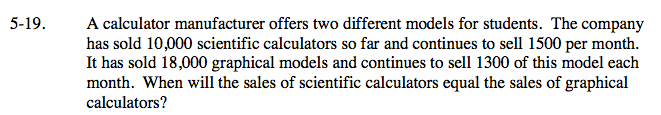Home > CAAC > Chapter 5 > Lesson 5.1.2 > Problem5-19

5-19.

A calculator manufacturer offers two different models for students. The company has sold 10,000 scientific calculators so far and continues to sell 1500 per month. It has sold 18,000 graphical models and continues to sell 1300 of this model each month. When will the sales of scientific calculators equal the sales of graphical calculators? 5-19 HW eTool (Desmos). Homework Help ✎First, define the variables.
Let x = time (months) and y = sales (\$)
Then, set up the two equations:

Scientific calculators: y = 10,000 + 1500x
Graphical calculators: Use an equation similar to the one above.

The sales will be equal when the equations are equal to each other. Use the Equal Values method.

Complete the tables in the eTool below to graph the results.
Click on the link at right for the full eTool version: AC 5-19 HW eTool